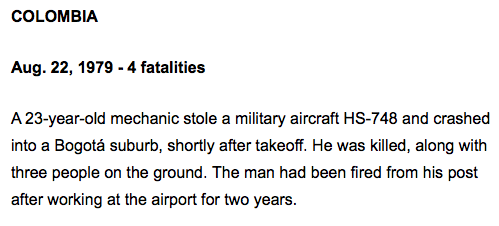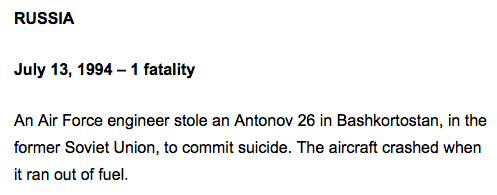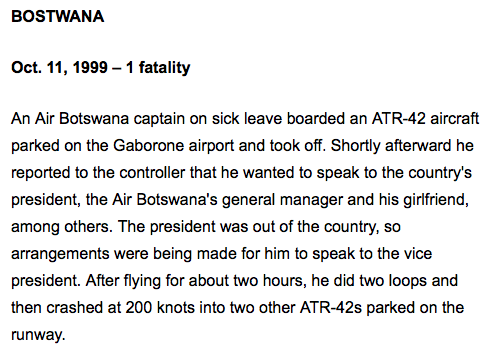## Thursday, March 26, 2015

### 76 | At Least Five 'Suicide by Pilot' Incidents Since 19769/26/76 = 9+26+76 = 1119/26/1976 = 9+2+6+1+9+7+6 = 408/22/79 = 8+22+79 = 1098/22/1978 = 8+2+2+1+9+7+9 = 387/13/94 = 7+30+94= 1147/13/1994 = 7+1+3+1+9+9+4 = 348/21/94 = 8+21+94 = 1238/21/1994 = 8+2+1+1+9+9+4 = 34Conspiracy = 12344 Fatalities....Kill = 11+9+12+12 = 44ATR-42... ATR = 1+20+18 = 3912/19/97 = 12+19+97 = 12812/19/1997 = 1+2+1+9+1+9+9+7 = 3910/11/99 = 10+11+99 = 12010/11/1999 = 1+0+1+1+1+9+9+9 = 31

Notice that these 'pilot suicide by plane incidents' began in the year '76, a number of much importance.  This is also the year that Ebola was discovered.

1.2.